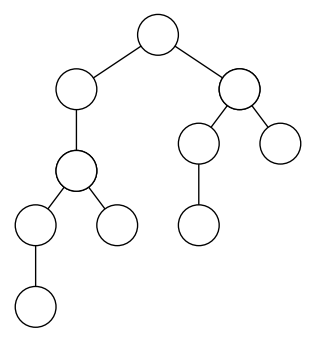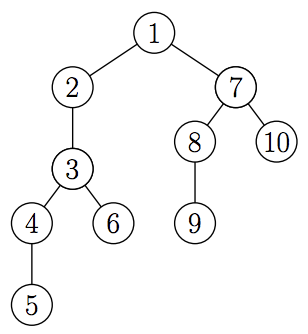시간 제한메모리 제한제출정답맞힌 사람정답 비율
7 초 512 MB133375.000%

## 문제

A fractal tree Fi is defined in the following way. First, a rooted tree F0 is given, which contains at least 2 vertices. Fi is then defined recursively in the following manner. Consider the set of vertices S which are leaves in Fi−1. For each vertex v in S, we replace it with a copy of F0, such that v corresponds to the root of F0.

Now, consider the tree Fk, for a given k. In this tree, we perform a depth-first search, where we visit all vertices of the tree recursively. At a certain vertex, we first recurse into the subtree of the leftmost child of the vertex, then the second leftmost child, and so on, until we have visited all the vertices in the subtree of the vertex. Assign integer labels to the vertices in the order they were visited, starting at 1. See Figure F.1 for an example.(a) The tree F0. (b) The tree F1. (c) The depth first search labelling of F1.

Given a set of queries consisting of pairs of vertices, your task is to find the distance between the two vertices. The distance is defined as the number of edges on the (unique) simple path between the two vertices.

## 입력

First, the tree F0 is given. The first line of input contains the number of vertices 2 ≤ n ≤ 100 000 in F0. The vertices are numbered 0 to n − 1, with 0 being the root vertex. Then follows a line containing n − 1 integers p1, . . . , pn−1. For each 1 ≤ i ≤ n − 1, the parent of node i in F0 is pi. It holds that pi < i. Within the tree, the left-to-right ordering of the vertices correspond to their numbering, in ascending order (i.e. the lowest-numbered child is the leftmost child).

The third line of input contains an integer 0 ≤ k < 230. Then follows a line containing an integer q, 1 ≤ q ≤ 100 000, the number of queries. Finally, there are q lines containing the queries. Each query is given by two distinct integers a and b, the labels of two vertices of Fk. You may assume that a and b are valid labels (i.e., they are between 1 and the number of vertices of Fk), and that they are at most 230.

## 출력

For each query (a, b), in the same order as given in the input, output the distance in Fk between the vertices labelled a and b.

## 예제 입력 1

4
0 1 0
1
10
1 2
1 4
1 6
1 8
1 10
5 10
6 8
9 3
7 10
8 9


## 예제 출력 1

1
3
3
2
2
6
5
5
1
1


## 출처• 문제를 만든 사람: Johan Sannemo